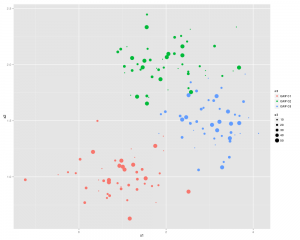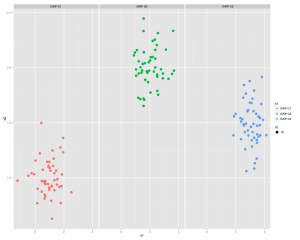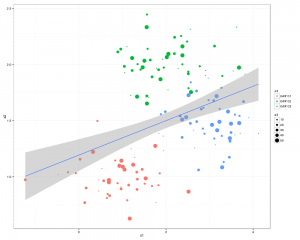# ggplot2/qplot basics

Install and load the ggplot2 and Cairo libraries

```install.packages(c("ggplot2","Cairo")
library(c(ggplot2,Cairo))
```

set up some data (or use some real data)

```x1<-rnorm(150,mean = rep(1:3, each =50),sd = 0.7)
x2<-rnorm(150,mean = rep(c(1,2,1.5), each = 50),sd = 0.2)
x3<-rnorm(150,mean = rep(c(20,30,3),each = 50)), sd = 0.5)
n3<-rep(c("GRP 01","GRP 02","GRP 03"),each=50)
```

Here is the command to generate the PNG file, with anti-aliasing:

```CairoPNG(filename = "Plot1.png", antialias="subpixel", width = 1000, height=800, units = "px")
{
qplot(x1,x2, ,color = n3, size = x3)
}
dev.off()
```or you can split the 3 sections up using:

` qplot(x1,x2, color = n3, facets = .~n3)`# ...and now something similar using GGPLOT2

First thing we need to do is create a dataframe from the four identical length vectors.

```df <- data.frame(x1,x2,x3,n3)
colnames(df) <- c("x1","x2","x3","n3")```

Some Charting:

```g1 <- ggplot(df,aes(x1,x2))
p <- g1 + geom_point(aes(colour=n3), size =3.5) +
geom_smooth(method = "lm") +
theme_bw()
print(p)
```

..and a slightly better looking version:

```g1 <- ggplot(df,aes(x1,x2))
p  <- g1 + geom_point(aes(colour=n3, size =x3)) +
geom_smooth(method = "lm") +
theme_bw()
print(p)
```There you go all good stuff.
Other things to check out: facet_wrap
Some more pretty graphics